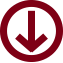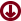C. elegans Protein Expression StudyFraction RAW data link CMS2 data link All fractionsFraction 1Fraction 2Fraction 3Fraction 4Fraction 5Fraction 6Fraction 7Fraction 8Fraction 9Fraction 10Fraction 11Fraction 12Fraction 15Fraction 16Unfractionated